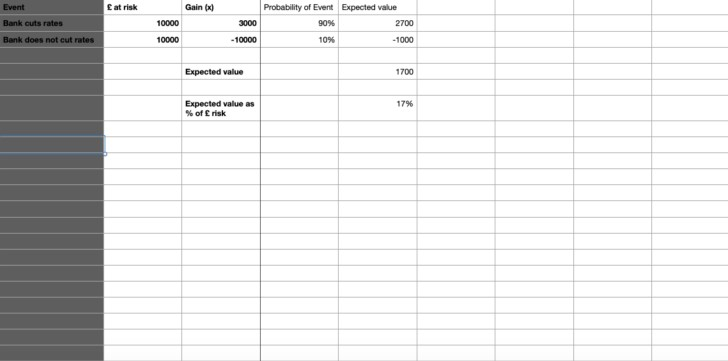# What you need to know!## Understanding the difference

For many traders Risk/Reward is the only trade management tool that matters. If the Risk/Reward is not greater than 1 then don’t take the trade. If the Risk/Reward (RR here on in) is low, say 0.5 the you should never take the trade. Don’t risk £10,000 to make £5,000 it never makes sense.

However, you may have found yourselves taking a trade where the RR is low, but you have high expectations the trade will work. Then you need to use something called expectancy. Expectancy is how you calculate whether a trade is worth taking.The way to calculate involves sitting down with a coffee and an uninterrupted 30 mins or so. Therefore,I have made a small video outlining how to do it which you can see here.

Here is the look of my spreadsheet below

Here are the formulas to use

Expectancy allows you to calculate whether a trade is worth taking even when the Risk/Reward is negative. The key to this is getting the probabilities of an event right. You can see in the table above that the risk reward is negative for the trade (only making 3000 for a risk of 10000). However, because the probability is high (90%) then the overall expectancy is positive at 17%. So, take 10 trades like this and you will be in the money overall.

So, this is why some traders trade with a negative RR at times. It is all about expectancy.